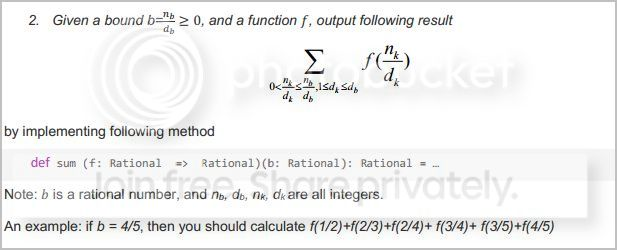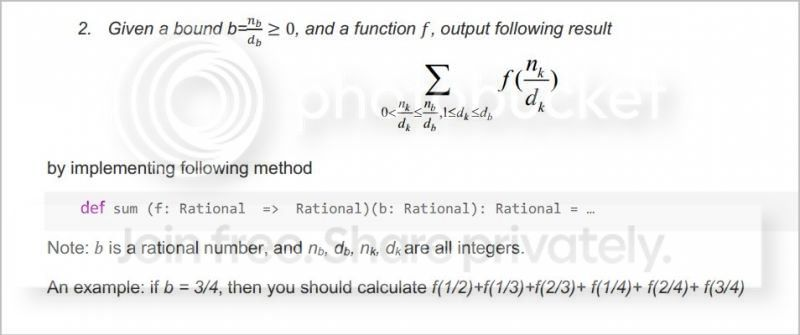programming forums Java Mobile Certification Databases Caching Books Engineering Micro Controllers OS Languages Paradigms IDEs Build Tools Frameworks Application Servers Open Source This Site Careers Other Pie Elite all forums
this forum made possible by our volunteer staff, including ...
Marshals:
• Campbell Ritchie
• Liutauras Vilda
• Tim Cooke
• Paul Clapham
• Jeanne Boyarsky
Sheriffs:
• Ron McLeod
• Frank Carver
• Junilu Lacar
Saloon Keepers:
• Stephan van Hulst
• Tim Moores
• Tim Holloway
• Al Hobbs
• Carey Brown
Bartenders:
• Piet Souris
• Frits Walraven
• fred rosenberger

# sum of rationals

Ranch Hand
Posts: 30
••Number of slices to send:
Optional 'thank-you' note:
••alright , so im trying to do this example in a book i found online. but im a little bit lost. heres a scan o f the problemnow , im still learning scala, but i went with what i have below , which doesnt work

and i already made my function > for the comparison. and the rational class. everything works ,so i just included the sum part only

MarshalPosts: 27371
88••Number of slices to send:
Optional 'thank-you' note:
••I'm lost too, because I don't understand the instructions. Nothing in the definition of the function says anything about the possible values of n-sub-k. So I don't see why f(1/3) and f(1/4) and f(2/5) and so on aren't part of the sum to be calculated. Perhaps something elsewhere in the book talks about that?John ortega
Ranch Hand
Posts: 30
••Number of slices to send:
Optional 'thank-you' note:
••Paul Clapham wrote:I'm lost too, because I don't understand the instructions. Nothing in the definition of the function says anything about the possible values of n-sub-k. So I don't see why f(1/3) and f(1/4) and f(2/5) and so on aren't part of the sum to be calculated. Perhaps something elsewhere in the book talks about that?

that is literally all it says lol , the question right above it asked to implement the divide function for rationals, which i did.

Paul Clapham
MarshalPosts: 27371
88••Number of slices to send:
Optional 'thank-you' note:
••Well, putting aside my griping about the details, what you need to do first is to write that summation in terms of some function of d-sub-b plus the same summation applied to d-sub-b-minus-1. The first part might also be a summation, and the termination condition of the second part happens when d-sub-b is 1.

I just spent a minute trying to do that, and I have to say that it looks a lot more difficult than division (although I haven't tried the functional programming version of division of fractions so I could be wrong). Anyway so far what you have doesn't look helpful to me, but I think that's because you started programming before you knew what your program should be doing. So slow down and figure out what needs to be done first, before you start typing any code.John ortega
Ranch Hand
Posts: 30
••Number of slices to send:
Optional 'thank-you' note:
••Paul Clapham wrote:Well, putting aside my griping about the details, what you need to do first is to write that summation in terms of some function of d-sub-b plus the same summation applied to d-sub-b-minus-1. The first part might also be a summation, and the termination condition of the second part happens when d-sub-b is 1.

I just spent a minute trying to do that, and I have to say that it looks a lot more difficult than division (although I haven't tried the functional programming version of division of fractions so I could be wrong). Anyway so far what you have doesn't look helpful to me, but I think that's because you started programming before you knew what your program should be doing. So slow down and figure out what needs to be done first, before you start typing any code.

yup your right, i had it in my head that this was a summation problem. but its looking like its something way different. I gotta try and understand what the heck this question is asking lol. also , im thinking that the example should go like this

An example: if b = 4/5, then you should calculate f(1/2)+f(1/3)+(f(2/3)+f(1/4)+f(2/4)+ f(3/4)+f(1/5)+f(2/5)+ f(3/5)+f(4/5)

is this a sum of rational numbers? im trying to figure out what it is the question is asking

Paul Clapham
MarshalPosts: 27371
88••Number of slices to send:
Optional 'thank-you' note:
••Well, yes it is a sum of rationals. (Can I assume you know how that capital-sigma sum thing works?) You've got a sum of things of the form f(n-sub-k/d-sub-k) for some function f, right? And you can see in the fragment of code that you're supposed to implement that the function f maps a Rational to a Rational. So its values are Rationals, and therefore your sum of things of the form f(n-sub-k/d-sub-k) is a sum of Rationals.John ortega
Ranch Hand
Posts: 30
••Number of slices to send:
Optional 'thank-you' note:
••yeah i understand capital sigma lol . darn , i thought i could go buy the sum of ints example i found online and thats the way i was goin about it. i guess that was wrong , how else can i go about this? im just starting with scala so im pretty much limited right now with what i could do lol

Paul Clapham
MarshalPosts: 27371
88••Number of slices to send:
Optional 'thank-you' note:
••John nava wrote:also , im thinking that the example should go like this

An example: if b = 4/5, then you should calculate f(1/2)+f(1/3)+f(2/3)+f(1/4)+f(2/4)+ f(3/4)+f(1/5)+f(2/5)+ f(3/5)+f(4/5)

Yeah, that's probably a good place to start from. My first try would be to split that into f(1/5)+f(2/5)+ f(3/5)+f(4/5), which are the addends which have d-sub-k = d-sub-b -- and f(1/2)+f(1/3)+f(2/3)+f(1/4)+f(2/4)+ f(3/4), which are the rest. The first part is a different recursive function, and the second is the same as the original summation only with d-sub-b reduced by 1 (the original was 5, but now you've reduced it to 4). I have to say I'm dubious that this process will work correctly though, I have the nagging feeling it's going to miss something. But it's a start anyway.John ortega
Ranch Hand
Posts: 30
••Number of slices to send:
Optional 'thank-you' note:
••Paul Clapham wrote:

John nava wrote:also , im thinking that the example should go like this

An example: if b = 4/5, then you should calculate f(1/2)+f(1/3)+f(2/3)+f(1/4)+f(2/4)+ f(3/4)+f(1/5)+f(2/5)+ f(3/5)+f(4/5)

Yeah, that's probably a good place to start from. My first try would be to split that into f(1/5)+f(2/5)+ f(3/5)+f(4/5), which are the addends which have d-sub-k = d-sub-b -- and f(1/2)+f(1/3)+f(2/3)+f(1/4)+f(2/4)+ f(3/4), which are the rest. The first part is a different recursive function, and the second is the same as the original summation only with d-sub-b reduced by 1 (the original was 5, but now you've reduced it to 4). I have to say I'm dubious that this process will work correctly though, I have the nagging feeling it's going to miss something. But it's a start anyway.

yeah the slides that im goin by that i found have an updated version of this question,i feel like this is gion way above me , im totally lost on what to do. i thought it would be easy to just plug in rationals into the sum of ints example i i found,, but apparently its muc more complexYour buns are mine! But you can have this tiny ad: Garden Master Course kickstarter https://coderanch.com/t/754577/Garden-Master-kickstarter